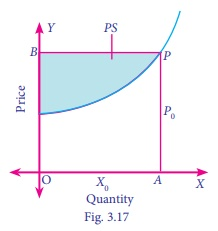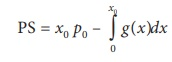Home | | Business Maths 12th Std | Integration: Producer surplus

# Integration: Producer surplus

A supply function g(x) represents the quantity that can be supplied at a price p.

Producer surplus

A supply function g(x) represents the quantity that can be supplied at a price p. Let p0 be the market price for the corresponding supply xo . But there can be some producers who are willing to supply the commodity below the market price gain from the fact that the price is p0. This gain is called the producerŌĆÖs surplus. It is represented in the following diagram.Mathematically, producerŌĆÖs surplus (PS) can be defined as,

PS = (Area of the rectangle OAPB) ŌłÆ (Area below the supply function from x = 0 to x = x0 )Example 3.28

Find the producerŌĆÖs surplus defined by the supply curve g(x) = 4x+8 when xo= 5.

Solution:= 140 ŌĆō (50 + 40)

= 50 units

Hence the producerŌĆÖs surplus= 50 units.

Example 3.29

The demand and supply function of a commodity are pd = 18 ŌłÆ 2x ŌłÆ x2 and ps = 2x ŌłÆ 3 . Find the consumerŌĆÖs surplus and producerŌĆÖs surplus at equilibrium price.

Solution:Hence at equilibrium price,

(i) the consumerŌĆÖs surplus is 27 units

(ii) the producerŌĆÖs surplus is 9 units.

Tags : Example Solved Problems with Answer, Solution, Formula , 12th Business Maths and Statistics : Chapter 3 : Integral Calculus - II
Study Material, Lecturing Notes, Assignment, Reference, Wiki description explanation, brief detail
12th Business Maths and Statistics : Chapter 3 : Integral Calculus - II : Integration: Producer surplus | Example Solved Problems with Answer, Solution, Formula